# Search Results (28)

View
Selected filters:
• MCCRS.Math.Content.7.G.A.2Rating

This lesson unit is intended to help you assess how well students are able to use geometric properties to solve problems. In particular, it will support you in identifying and helping students who have the following difficulties: Solving problems relating to using the measures of the interior angles of polygons; and solving problems relating to using the measures of the exterior angles of polygons.

Subject:
Geometry
Material Type:
Assessment
Lesson Plan
Provider:
Shell Center for Mathematical Education
U.C. Berkeley
Provider Set:
Mathematics Assessment Project (MAP)
Mathematics Assessment Project (MAP)
04/26/2013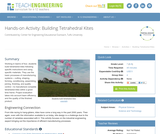Conditions of Use:
Rating

Working in teams of four, students build tetrahedral kites following specific instructions and using specific materials. They use the basic processes of manufacturing systems – cutting, shaping, forming, conditioning, assembling, joining, finishing, and quality control – to manufacture complete tetrahedral kites within a given time frame. Project evaluation takes into account team efficiency and the quality of the finished product.

Subject:
Engineering
Material Type:
Activity/Lab
Provider:
TeachEngineering
Provider Set:
TeachEngineering
09/18/2014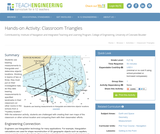Conditions of Use:
Rating

Students use bearing measurements to triangulate and determine objects' locations. Working in teams of two or three, they must put on their investigative hats as they take bearing measurements to specified landmarks in their classroom (or other rooms in the school) from a "mystery location." With the extension activity, students are challenged with creating their own maps of the classroom or other school location and comparing them with their classmates' efforts.

Subject:
Education
Material Type:
Activity/Lab
Provider:
TeachEngineering
Provider Set:
TeachEngineering
Author:
Janet Yowell
Malinda Schaefer Zarske
Matt Lippis
10/14/2015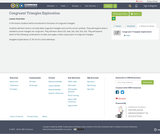Conditions of Use:
Remix and Share
Rating

In this lesson students will be introduced to the basics of congruent triangles.Students will learn how to correctly label congruent triangles and use the correct symbols. They will explore what is needed to prove triangles are congruent. They will learn about SSS, AAA, SAS, ASA, SSA, SAS. They will explore which of the following combinations of sides and angles create unique pairs of congruent triangles. Geogebra Explorations CC BY SA 4.0 Lorena Mendoza

Subject:
Mathematics
Material Type:
Lesson Plan
Author:
Julie Stoker
04/27/2018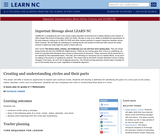Conditions of Use:
Remix and Share
Rating

This lesson will offer a hands-on opportunity to explore and construct circles. Students will develop a definition for identifying the parts of a circle such as the center, radius, diameter, chord, and circumference. Students will use compasses and rulers in constructing these parts of a circle.

Subject:
Geometry
Material Type:
Lesson Plan
Provider:
University of North Carolina at Chapel Hill School of Education
Provider Set:
LEARN NC Lesson Plans
Author:
Patricia Tingen
06/25/1999Rating

This lesson unit is intended to help assess how well students are able to interpret and use scale drawings to plan a garden layout. This involves using proportional reasoning and metric units.

Subject:
Algebra
Geometry
Ratios and Proportions
Material Type:
Assessment
Lesson Plan
Provider:
Shell Center for Mathematical Education
U.C. Berkeley
Provider Set:
Mathematics Assessment Project (MAP)
Mathematics Assessment Project (MAP)
04/26/2013Conditions of Use:
Rating

Students use simple materials to design an open spectrograph so they can calculate the angle light is bent when it passes through a holographic diffraction grating. A holographic diffraction grating acts like a prism, showing the visual components of light. After finding the desired angles, students use what they have learned to design their own spectrograph enclosure.

Subject:
Engineering
Physics
Material Type:
Activity/Lab
Provider:
TeachEngineering
Provider Set:
TeachEngineering
10/14/2015Rating

This lesson unit is intended to help you assess how well students are able to: solve simple problems involving ratio and direct proportion; choose an appropriate sampling method; and collect discrete data and record them using a frequency table.

Subject:
Education
Geometry
Measurement and Data
Ratios and Proportions
Material Type:
Assessment
Lecture Notes
Lesson Plan
Teaching/Learning Strategy
Provider:
Florida Center for Research in Science, Technology, Engineering, and Mathematics
Shell Center for Mathematical Education
U.C. Berkeley
Provider Set:
iCPALMS: A Standards-based K-12 Resources and Tools Pathway
Mathematics Assessment Project (MAP)
Mathematics Assessment Project (MAP)
Author:
http://map.mathshell.org/
04/26/2013Rating

This lesson unit is intended to help you assess how well students are able to: Model a situation; make sensible, realistic assumptions and estimates; and use assumptions and estimates to create a chain of reasoning, in order to solve a practical problem.

Subject:
Geometry
Material Type:
Assessment
Lesson Plan
Provider:
Shell Center for Mathematical Education
U.C. Berkeley
Provider Set:
Mathematics Assessment Project (MAP)
Mathematics Assessment Project (MAP)
04/26/2013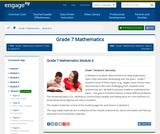Conditions of Use:
Remix and Share
Rating

In Module 6, students delve further into several geometry topics they have been developing over the years.  Grade 7 presents some of these topics, (e.g., angles, area, surface area, and volume) in the most challenging form students have experienced yet.  Module 6 assumes students understand the basics.  The goal is to build a fluency in these difficult problems.  The remaining topics, (i.e., working on constructing triangles and taking slices (or cross-sections) of three-dimensional figures) are new to students.

Subject:
Geometry
Material Type:
Module
Provider:
New York State Education Department
Provider Set:
EngageNY
02/02/2014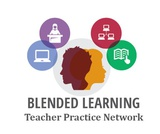Conditions of Use:
Remix and Share
Rating

Throughout this problem-based learning module students will address real world skills. Students will be asked to brainstorm ideas and think innovatively both independently and collaboratively in addressing a real-world problem that is relevant to their daily lives and surroundings.  Students/teams will be encouraged to use the internet for research purposes in their design phase. What components should be included for a modern, updated classroom? Students will utilize various online platforms to design an ideal, modern, 21st century “dream classroom”.  Students will incorporate components that would meet the needs of all learners and a classroom that would be able to integrate technology. These classrooms can be shared with relevant individuals in the community and others in the school building.

Subject:
Mathematics
Material Type:
Lesson Plan
Author:
Blended Learning Teacher Practice Network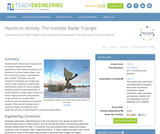Conditions of Use:
Rating

Students learn about radar imaging and its various military and civilian applications that include recognition and detection of human-made targets, and the monitoring of space, deforestation and oil spills. They learn how the concepts of similarity and scaling are used in radar imaging to create three-dimensional models of various targets. Students apply the critical attributes of similar figures to create scale models of a radar imaging scenario using infrared range sensors (to emulate radar functions) and toy airplanes (to emulate targets). They use technology tools to measure angles and distances, and relate the concept of similar figures to real-world applications.

Subject:
Education
Material Type:
Activity/Lab
Provider:
TeachEngineering
Provider Set:
TeachEngineering
Author:
Mounir Ben Ghalia
Rocio Denise Nava
10/14/2015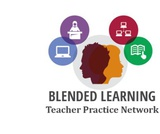Conditions of Use:
Remix and Share
Rating

In this problem-based learning module, students will work collaboratively to improve the accessibility or safety of their school or community. For example, students could identify that accessibility ramps need to be added to the school property or additional sidewalks need to be created/repaired to increase the safety of students as they walk to school. Students would work together to create models of these improvements and create a communications plan that informs the stakeholders of the materials needed to create these improvements (i.e. using volume to determine the amount of concrete, using angles to determine measurements for ramps, etc..).

Subject:
Geometry
Material Type:
Lesson Plan
Author:
Blended Learning Teacher Practice Network
11/21/2017Conditions of Use:
Remix and Share
Rating

Constructions and Angles

Unit Overview

Type of Unit: Concept

Prior Knowledge

Students should be able to:

Use a protractor and ruler.
Identify different types of triangles and quadrilaterals and their characteristics.

Lesson Flow

After an initial exploratory lesson involving a paper folding activity that gets students thinking in general about angles and figures in a context, the unit is divided into two concept development sections. The first section focuses on types of angles—adjacent, supplementary, complementary, and vertical—and how they are manifested in quadrilaterals. The second section looks at triangles and their properties, including the angle sum, and how this affects other figures.

In the first set of conceptual lessons, students explore different types of angles and where the types of angles appear in quadrilaterals. Students fold paper and observe the angles formed, draw given angles, and explore interactive sketches that test many cases. Students use a protractor and ruler to draw parallelograms with given properties. They explore sketches of parallelograms with specific properties, such as perpendicular diagonals. After concluding the investigation of the angle types, students move on to the next set.

In the second set of conceptual development lessons, students focus on triangles. Students again fold paper to create figures and certain angles, such as complementary angles.

Students draw, using a protractor and ruler, other triangles with given properties. Students then explore triangles with certain known and unknown elements, such as the number of given sides and angles. This process starts with paper folding and drawing and continues with exploration of interactive sketches. Students draw conclusions about which cases allow 0, 1, 2, or an infinite number of triangles. In the course of the exploration, students discover that the sum of the measure of the interior angles of a triangle is 180°. They also learn that the sum of the measures of the interior angles of a quadrilateral is 360°. They explore other polygons to find their angle sum and determine if there is a relationship to angle sum of triangles. The exploration concludes with finding the measure of the interior angles of regular polygons and speculating about how this relates to a circle.

Lastly, students solve equations to find unknown angle measures. Using their previous experience, students find the remaining angle measures in a parallelogram when only one angle measure is given. Students also play a game similar to 20 Questions to identify types of triangles and quadrilaterals. Having completed the remaining lessons, students have a four-day Gallery to explore a variety of problems.

The unit ends with a unit assessment.

Subject:
Mathematics
Geometry
Material Type:
Unit of Study
Provider:
Pearson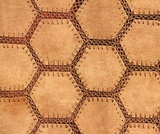Conditions of Use:
Remix and Share
Rating

Students learn more about the characteristics of parallelograms by folding paper and measuring the angles in a parallelogram. Students use a ruler and protractor to draw parallelograms with given properties. Then, students use a ruler and protractor to draw a rectangle.Key ConceptsOpposite angles of a parallelogram are congruent.Consecutive angles of a parallelogram are supplementary.Diagonals of a parallelogram bisect each other.Diagonals of a rectangle are congruent.Goals and Learning ObjectivesAccess prior knowledge of parallelograms.Understand that the sum of angle measures in any quadrilateral is 360°.Understand the relationship of the angles and diagonals in a parallelogram.Understand the relationship of the angles and diagonals in a rectangle.

Subject:
Geometry
Material Type:
Lesson Plan
Provider:
Pearson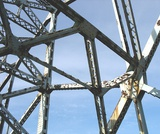Conditions of Use:
Remix and Share
Rating

Students learn to classify triangles by the size of the angles and the measures of the sides.Key ConceptsTriangles are polygons with three sides.Scalene triangles have all sides with a different length and all angles with a different measure.Isosceles triangles have two sides with the same length and two angles with the same measure.Equilateral triangles have all sides with the same length and all angles with the same measure.Acute triangles have all angles with a measure less than 90°.Obtuse triangles have one angle with a measure greater than 90°.Right triangles have one angle with a measure of 90°.ELL: Keep in mind that consistency at the beginning is very important as students begin to learn and apply math vocabulary.Goals and Learning ObjectivesExplore conditions that result in triangles.Identify types of triangles based on the measure of the angles or the measures of the sides.

Subject:
Geometry
Material Type:
Lesson Plan
Provider:
Pearson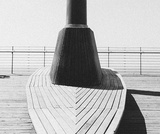Conditions of Use:
Remix and Share
Rating

Students learn how the diagonals of a rhombus are related. They use interactive sketches to learn about the properties of the angles and diagonals of squares, rectangles, rhombuses, parallelograms, and other quadrilaterals.Key ConceptsThe sum of the measures of the angles of all quadrilaterals is 360°.The alternate angles (nonadjacent angles) of rhombuses and parallelograms have the same measure.The measure of the angles of rectangles and squares is 90°.The consecutive angles of parallelograms and rhombuses are supplementary. This applies to squares and rectangles as well.The diagonals of a parallelogram bisect each other.The diagonals of a rectangle are congruent and bisect each other.The diagonals of a rhombus bisect each other and are perpendicular.Goals and Learning ObjectivesMeasure the angles formed by the intersection of the diagonals of a rhombus.Explore the relationships of the angles of different squares, rectangles, rhombuses, parallelograms, and other quadrilaterals.Explore the relationships of the diagonals of different squares, rectangles, rhombuses, parallelograms, and other quadrilaterals.

Subject:
Geometry
Material Type:
Lesson Plan
Provider:
Pearson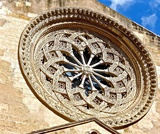Conditions of Use:
Remix and Share
Rating

Lesson OverviewStudents explore different polygons by drawing them, measuring angles, and manipulating interactive sketches to find the angle sum for any polygon. Students also explore the angle measures in regular polygons.Key ConceptsThe angle sum in a triangle is 180°. A quadrilateral can be composed of two triangles, so the angle sum of a quadrilateral is 360°.The number of triangles that compose a polygon is two less than the number of sides (angles). The sum of the interior angles in a polygon with n sides is 180° (n – 2).Goals and Learning ObjectivesFind angle sums in polygons.Generalize to find the angle sum for any polygon.Find interior angle measures for regular polygons.

Subject:
Geometry
Material Type:
Lesson Plan
Provider:
Pearson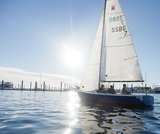Conditions of Use:
Remix and Share
Rating

Students explore properties of triangles. They fold paper to make a triangle and measure its angles. Students also draw triangles with given conditions.Key ConceptsThe sum of the measures of the angles in a triangle is always 180°.Given different side measures and/or angle measures, some scenarios will create triangles and others will not. Students explore various cases and draw conclusions about which conditions create triangles and why.Goals and Learning ObjectivesDraw triangles with given conditions.Find the sum of the measures of the angles of a triangle.Explore conditions that result in triangles.

Subject:
Geometry
Material Type:
Lesson Plan
Provider:
Pearson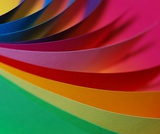Conditions of Use:
Remix and Share
Rating

Students discuss what they know about shapes and their characteristics through a paper-folding activity that results in a parallelogram.Key ConceptsQuadrilaterals and triangles are classified by their different characteristics; the types of angles and sides define the shapes. While students are familiar with some of the characteristics of these shapes, they begin to explore other aspects of theses figures. Students review what they know about these shapes so far.Goals and Learning ObjectivesReview characteristics that describe quadrilaterals and triangles.Discuss what students know about these shapes.Explore other aspects of these shapes.

Subject:
Geometry
Material Type:
Lesson Plan
Provider:
Pearson